Gyro.mws

Gyroscope

We visualize the motion of the simple gyroscope discussed in the text.

The objective is to explain how Euler's equations for the angular velocity vector which defines the motion of the gyroscope are giving this quantity in terms of components in the (almost) body frame (a frame in which the moments of inertia don't change, and in which the external gravitational torque is still easily defined). The task is then to connect these components to the laboratory frame. This is done through two rotation angles, theta and phi, as explained in Example 13.7 in J.M. Knudsen and P.G. Hjorth: Elements of Newtonian Mechanics .

This version was corrected for a previous error, and is up to date as of April 12, 2004.

An interesting extension for this worksheet would be the introduction of frictional torques to explain phenomena such as the sleeper mode for a spinning top.

 > restart: with(linalg):

```Warning, the protected names norm and trace have been redefined and unprotected

```

We begin with the full angular momentum vector (bottom of page 338):

 > Lv:=vector([-diff(theta(t),t)*I1,-diff(phi(t),t)*sin(theta(t))*I2,(diff(phi(t),t)*cos(theta(t))+w0)*I3]);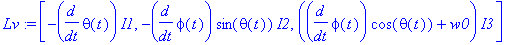The angular velocity vector (big-Omega with the spin removed): it defines the 'almost body frame"

 > Wv:=vector([-diff(theta(t),t),-diff(phi(t),t)*sin(theta(t)),diff(phi(t),t)*cos(theta(t))]);The rate of change of the angular momentum vector is given by the cross product of Omega  and L  (page 339) plus the rate of change in the 'almost body frame'.

Get the cross product:

 > WcrL:=map(simplify,crossprod(Wv,Lv));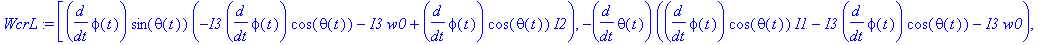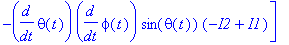We define a vector with the time derivative of angular momentum in the non-inertial 'almost-body' frame:

 > Ldotv:=map(expand,map(diff,op(Lv),t));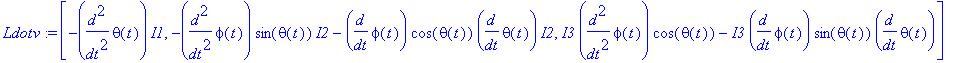Now we have the total rate of change of L  ( ( dL/dt|_lab  which is equal to the torque) as expressed in unit vectors of the (almost) body frame:

 > LdotS:=map(simplify,evalm(Ldotv+WcrL));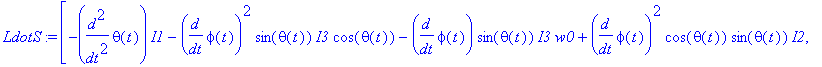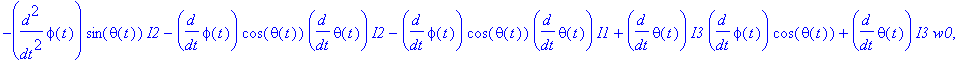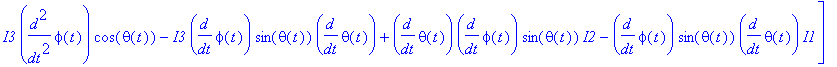Instead of Euler's equations we apply directly the angular momentum theorem: the gravitational torque is always about the x  (1-) axis:

 > eq1:=LdotS=-M*g*l*sin(theta(t));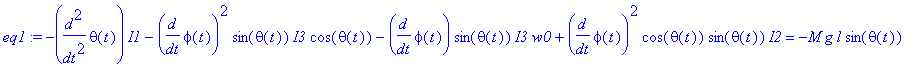> eq2:=LdotS=0;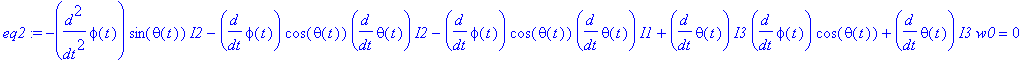> eq3:=LdotS=0;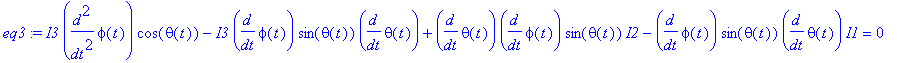Now we insert some values for the moments of inertia, etc:

 > l:=1: g:=10: M:=1: I1:=1/4+1: I2:=I1: I3:=1/2: w0:=20:

Initial condition: a horizontal gyro aligned with the x -axis in the lab and being let go.

 > th0:=Pi/2: ph0:=0: thdot0:=0: phidot0:=0:

The pair eq1/eq3  doesn't work! This is because eq3  represents just conservation of the 3-component of angular momentum:

 > eq3;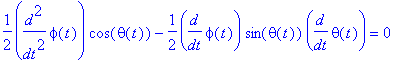> t:='t': sol:=dsolve({eq1,eq2,theta(0)=th0,D(theta)(0)=thdot0,phi(0)=ph0,D(phi)(0)=phidot0},{theta(t),phi(t)},numeric,method=gear,output=listprocedure);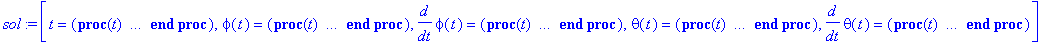> Theta:=subs(sol,theta(t)): Phi:=subs(sol,phi(t)):

 > plot(['Theta(t)','Phi(t)'],t=0..20,color=[blue,green],numpoints=1000,thickness=3);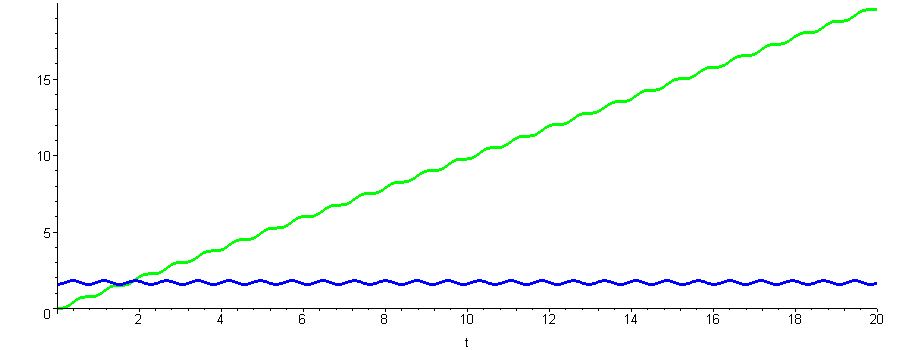The green curve ( phi ) describes precession, while the oscillation in theta (blue curve) corresponds to nutation about some average value (determined by the initial conditions).

 > plot('Theta(t)',t=0..4,numpoints=1000,thickness=3);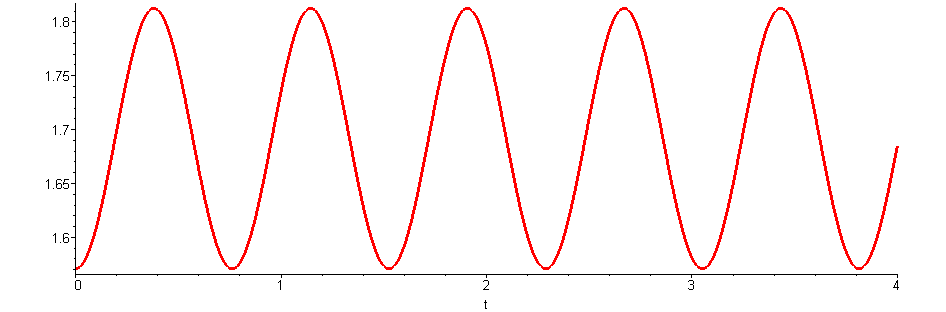For the plot of the gyro we need to connect to the laboratory frame. We simply define the Cartesian components of the position vector in terms of arm length l, polar (nutation) angle theta, and azimuthal (precession) angle phi.

 > with(plottools):

We record an animation, i.e., we define a time sequence of 3d plots, and use the arrow command to depict the omega-vector in the lab frame.

We use the numerical solution available for theta(t)  and phi(t)  to construct the time evolution of the vector representing the gyro axis.

 > N:=300: for i from 1 to N do: t:=0.03*i: Prec:=vector([l*sin(Theta(t))*cos(Phi(t)),l*sin(Theta(t))*sin(Phi(t)),l*cos(Theta(t))]) ; ll[i] := arrow(vector([0,0,0]), Prec, .2, .4, .1): od:

 > i:='i': plots[display3d]([seq(ll[i],i=1..N)], color=red,orientation=[66,48],axes=boxed,insequence=true);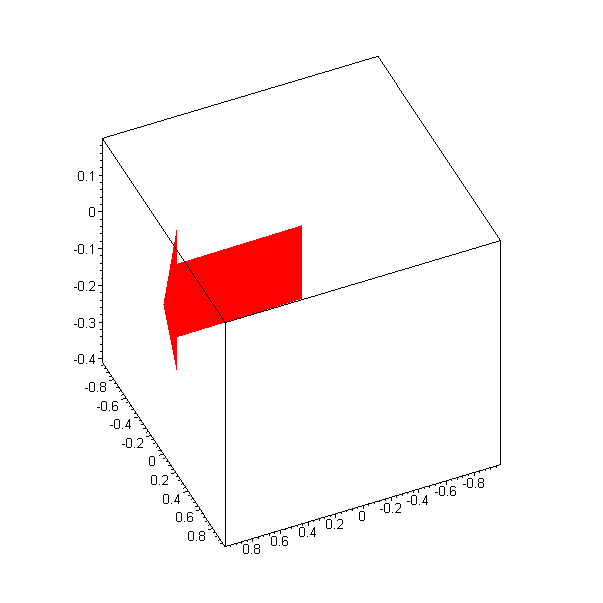Exercise 1:

Change the initial conditions and obtain different motion: e.g., by pushing the gyro into pure precession without nutation. What happens when you reverse the initial push?

Exercise 2:

Consider a torque free gyroscope. Start the motion with a push in the nutational direction (hammer blow on the gyro axle). Observe the motion. You can make the gyro torque-free by setting g=0 .

 >

 >

 >

 >

 >

 >

 >

 >

 >

 >

 >